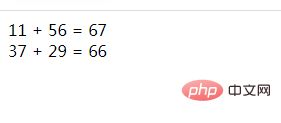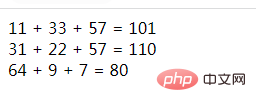# php函数的参数赋值有哪几种

php函数的参数赋值有3种：1、值传递赋值，将实参的值复制一份再赋值给函数的形参；2、引用传递赋值，把实参的内存地址复制一份，然后传递给函数的形参，进而将实参值赋值给形参；3、直接给函数的参数指定默认值，语法“函数名(参数变量='值')”。php函数的参数赋值有3种：

1、值传递赋值

2、引用传递赋值

3、默认参数值

1、值传递赋值

```<?php
function swap(\$a, \$b){
echo '函数内，交换前 \$a = '.\$a.', \$b = '.\$b.'<br>';
\$temp = \$a;
\$a = \$b;
\$b = \$temp;
echo '函数内，交换后 \$a = '.\$a.', \$b = '.\$b.'<br>';
}
\$x = 5;
\$y = 7;
echo '函数外，交换前 \$x = '.\$x.', \$y = '.\$y.'<br>';
swap(\$x, \$y);
echo '函数外，交换后 \$x = '.\$x.', \$y = '.\$y;
?>```2、引用传递赋值

```function name (&参数1, &参数2, ..., &参数3) {
...
}```

```<?php
function swap(&\$a, &\$b){
echo '函数内，交换前 \$a = '.\$a.', \$b = '.\$b.'<br>';
\$temp = \$a;
\$a = \$b;
\$b = \$temp;
echo '函数内，交换后 \$a = '.\$a.', \$b = '.\$b.'<br>';
}
\$x = 5;
\$y = 7;
echo '函数外，交换前 \$x = '.\$x.', \$y = '.\$y.'<br>';
swap(\$x, \$y);
echo '函数外，交换后 \$x = '.\$x.', \$y = '.\$y;
?>```3、默认参数值

```function name (\$str = '默认值', \$url) {
echo \$str;
}```

```<?php
echo \$a.' + '.\$b.' = '.(\$a+\$b).'<br>';
}
?>``````<?php
echo \$a.' + '.\$b.' + '.\$c.' = '.(\$a+\$b+\$c).'<br>';
}
?>```#### 相关文章推荐

• php怎么根据年月日判断是一年的第几天• php怎么查找字符串是第几位• php怎么将string转为日期格式• php怎么去掉多个相同字符• php怎么检查汉字字符串有几个字符
1/1